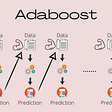# How can I design a PID Controller to stabllize the plant 1/(s³+1) ?

I have a system, it’s Transfer function is :

1

— — — — — —

s³ + 1

and I want to design a Discrete PID Controller so discretized the plant at sampling time 0.05s :

0.000019z² + 0.00008z + 0.000021

— — — — — — — — — — — — — — — — — — — — — — — — — (Sorry, it is not clean..)

z³ -2.99994z² + 3.00006z — 1

This system is unstable and Discrete PID Controller Block’s autotuner cannot find Kp, Ki, Kd to stablize the system.

I want to design a PID Controller, not another Controller.

I think it should be added such a filter, derivative filter(Differtiator?) or a Integrator…

How can I design the PID Controller in Matlab and Simulink? It can be designed?

NOTE:-

Matlabsolutions.com provide latest MatLab Homework Help,MatLab Assignment Help for students, engineers and researchers in Multiple Branches like ECE, EEE, CSE, Mechanical, Civil with 100% output.Matlab Code for B.E, B.Tech,M.E,M.Tech, Ph.D. Scholars with 100% privacy guaranteed. Get MATLAB projects with source code for your learning and research.

o you have a transfer function G(s) = 1/(s³+1) and you want to find gains P,I,D for your controller K(s) = P+D*s+I/s. As commonly known, the closed loop transfer function T(s) can be written as;

T(s) = K(s)*G(s)/(1+G(s)*K(s))

If you plugin G(s) and K(s) functions, you will obtain;

T(s) = (D*s² + P*s + I) / (s⁴ + D*s² + (P+1)*s + I)

From here, it should be clear that a simple controller, K(s) = P+D*s+I/s, cannot stabilize the system (hint: routh-hurwitz stability criterion). That’s why your controller needs to have a term that will show up as the s³ term.

One option is to add a C*s² term to your controller. This way your K(s) = P+D*s+I/s+C*s², and resulting closed loop transfer function is;

T(s) = (C*s³ + D*s² + P*s + I)/( s⁴ + C*s³ + D*s² + (P+1)*s + I )

For instance, imagine you want to place your poles to (-4+0i) (continous time model), then your characteristic equation needs to be;

(s+4)⁴ = s⁴ + 16*s³ + +96*s² + 256*s + 256 = ( s⁴ + C*s³ + D*s² + (P+1)*s + I )

From here, by matching the coefficients, you can find the controller

--

--

--

## More from Technical Source

Simple! That is me, a simple person. I am passionate about knowledge and reading. That’s why I have decided to write and share a bit of my life and thoughts to.

Love podcasts or audiobooks? Learn on the go with our new app.

## Installing a Webserver & Testing a Webpage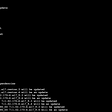## Prototyping Next Generation Customer Experiences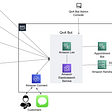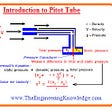## Present Simple## My Experience Volunteering with Hamoye## Grid layout, grid layout everywhere!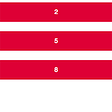## Technical Source

Simple! That is me, a simple person. I am passionate about knowledge and reading. That’s why I have decided to write and share a bit of my life and thoughts to.

## A dive into what is Multiple Linear Regression and Why should I care?